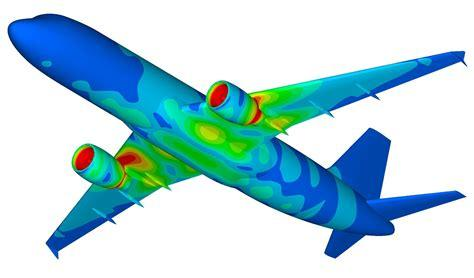一、有限元能干什么

## 一、有限元能干什么## 二、偏微分方程如何求解（有限元发明以前）曾攀-有限元分析基础教程

## 四、有限元法的数学基础——降维MATLAB代码如下：

function M = MassMat1D(x)
n = length(x)-1; % number of subintervals
M = zeros(n+1,n+1); % allocate mass matrix
for i = 1:n % loop over subintervals
h = x(i+1) - x(i); % interval length
M(i,i) = M(i,i) + h/3; % add h/3 to M(i,i)
M(i,i+1) = M(i,i+1) + h/6;
M(i+1,i) = M(i+1,i) + h/6;
M(i+1,i+1) = M(i+1,i+1) + h/3;
endMATLAB代码如下：

function b = LoadVec1D(x,f)
n = length(x)-1;
b = zeros(n+1,1);
for i = 1:n
h = x(i+1) - x(i);
b(i) = b(i) + f(x(i))*h/2;
b(i+1) = b(i+1) + f(x(i+1))*h/2;
end

function y = Foo(x)
y=x.*sin(x)

n = 5 ;% number of subintervals
h = 1/n; % mesh size
x = 0:h:1; % mesh
y = Foo(x);% calc f(x)
M = MassMat1D(x); % assemble mass
Pf = M\b; % solve linear system
plot(x,Pf,x,y); % plot  projection

 Larson, Mats G., and Fredrik Bengzon. "The finite element method: theory, implementation, and practice."

## 五、如何获得“弱形式”的解为二阶无穷小，可略去，所以可以得到：为边界条件带来的等效矩阵，显然只有或者才有取值，很容易求得，即MATLAB代码如下：

function A = StiffMat1D(x,kappa)
n = length(x)-1;
A = zeros(n+1,n+1);
for i = 1:n
h = x(i+1) - x(i);
A(i,i) = A(i,i) + 1/h;
A(i,i+1) = A(i,i+1) - 1/h;
A(i+1,i) = A(i+1,i) - 1/h;
A(i+1,i+1) = A(i+1,i+1) + 1/h;
end
A(1,1) = A(1,1) + kappa(1);
A(n+1,n+1) = A(n+1,n+1) + kappa(2);MATLAB代码如下：

function b = LoadVec1D(x,f,kappa,g)
n = length(x)-1;
b = zeros(n+1,1);
for i = 1:n
h = x(i+1) - x(i);
b(i) = b(i) + f(x(i))*h/2;
b(i+1) = b(i+1) + f(x(i+1))*h/2;
end
b(1) = b(1) + kappa(1)*g(1);
b(n+1) = b(n+1) + kappa(2)*g(2);function y = Source(x)
y = 0.03*(x-6)ˆ4; % heat source

%%solve Poission equations
h = 0.1; % mesh size
x = 2:h:8; % mesh
kappa = [1.e+6 0];
g = [-1 0];
A = StiffMat1D(x, kappa);
b = LoadVec1D(x, @Source, kappa, g);
U = A\b;
plot(x,U)

 Larson, Mats G., and Fredrik Bengzon. "The finite element method: theory, implementation, and practice."

## 六、二维、三维有限元计算